Most of us would be familiar with the 16×2 Dot matrix LCD display that is used in most of the projects to display some information to the user.  But these LCD displays have a lot of limitations. In this tutorial, we are going to learn about OLED display and how to use them with Raspberry Pi. There are lots of types of OLED displays available in the market and there are lots of ways to get them working. We have already used 7 Pin OLED with Arduino.

### Hardware Required:

• 128×64 OLED display Module (SSD1306)
• Raspberry Pi
• Connecting Wires
• Power supply

### Getting to know about OLED Displays:

The term OLED stands for “Organic Light emitting diode” it uses the same technology that is used in most of our televisions but has fewer pixels compared to them.  It is real fun to have these cool looking display modules to be interfaced with the Raspberry Pi since it will make our projects look cool. We have covered a full Article on OLED displays and its types here. Here, we are using a Monochrome 4-pin SSD1306 0.96” OLED display. This LCD can only work with the I2C mode.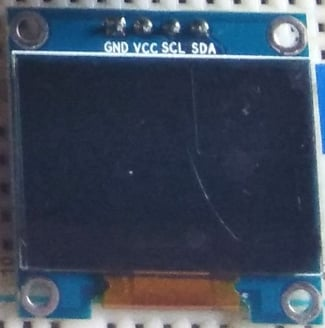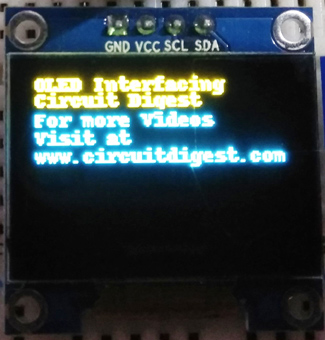Below are the connections of OLED with Raspberry pi:​

 OLED Pin RPI Pin VCC 3.3v GND GND SDA SDA (Physical pin 3) SCL SCL (Physical pin 5)

### Circuit Diagram: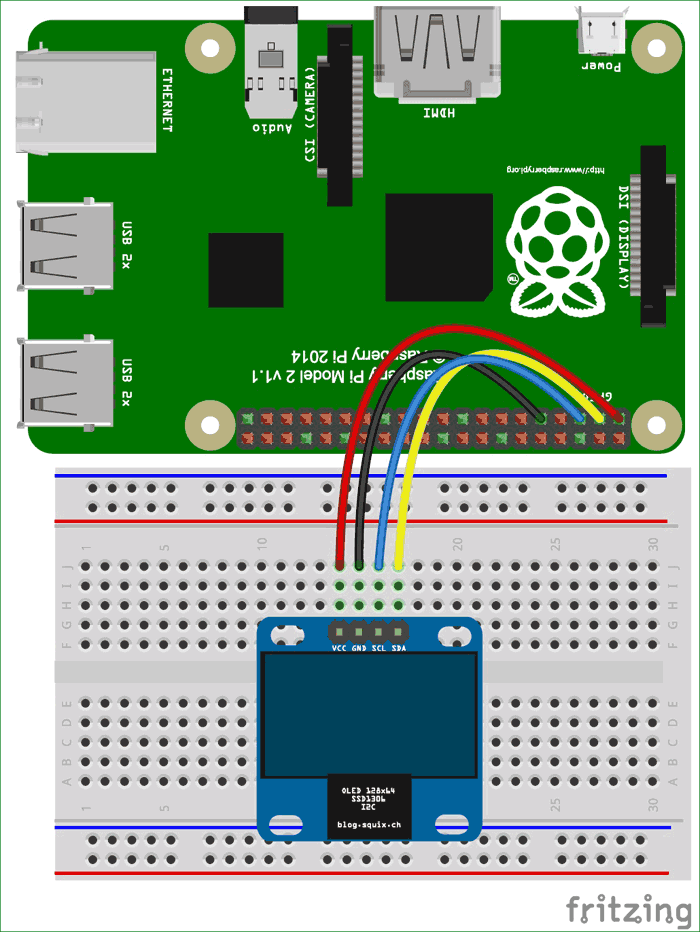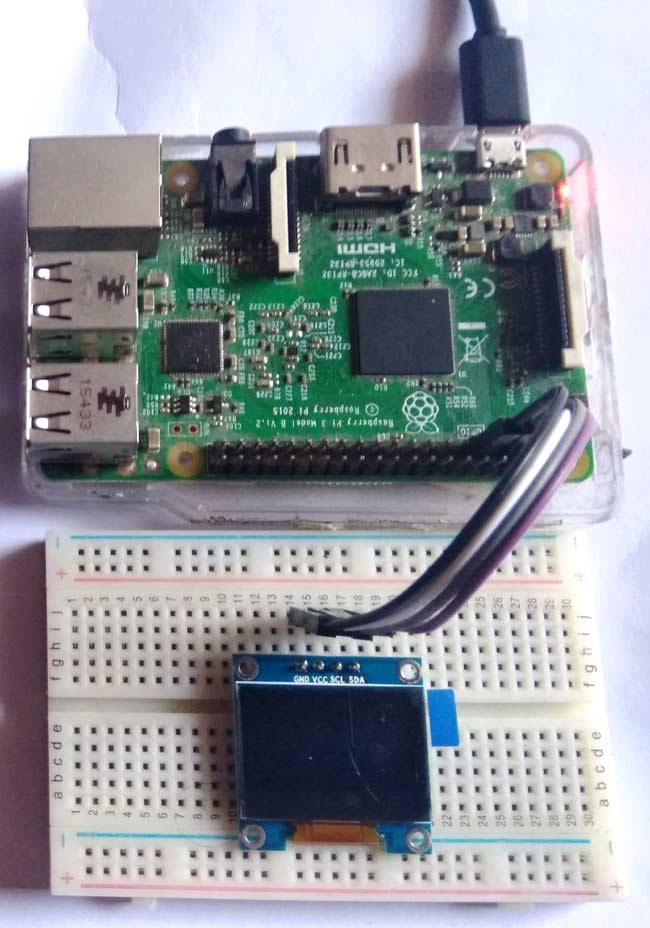### Connecting OLED with Raspberry Pi:

RPI community has already given us a lot of Libraries which can be directly used to make this a lot simpler. I tried out a few libraries and found that the Adafruit_SSD1306 OLED Library was very easy to use and has a handful of graphical options hence we will use the same in this tutorial.

Step 1: Enable I2C communication

Before installing Adafruit SSD1306 library we need to enable I2C communication in Raspberry Pi.

To do this type in Raspberry Pi console:

`sudo raspi-config`

And then a blue screen will appear. Now select interface option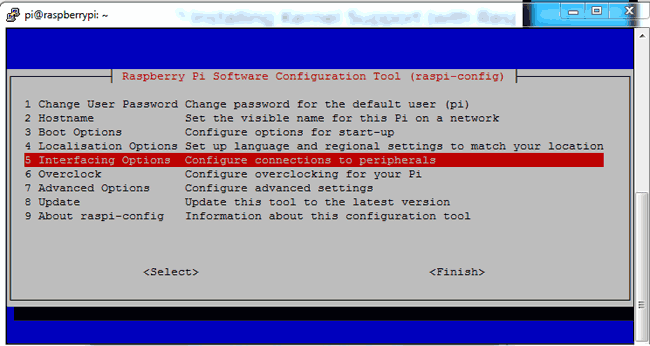After this, we need to need to select I2C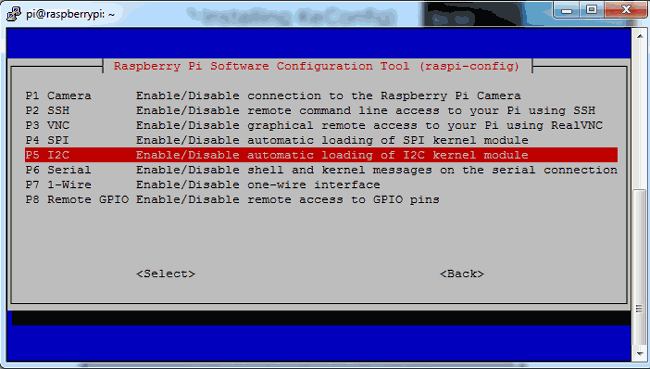After this, we need to select yes and press enter and then ok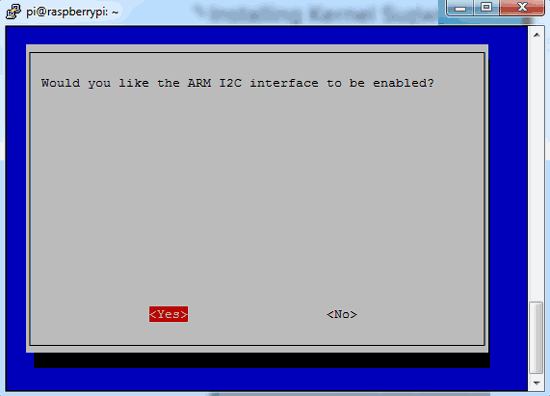After this, we need to reboot raspberry pi by issuing below command:

`sodo reboot`

Step 2: Find OLED I2C address and update it

Then we need to find OLED I2C address by using given command and you will see a hex address.

`sudo i2cdetect –y 1`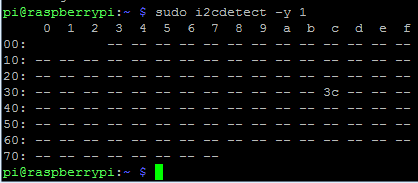Then update Raspberry Pi using given command:

`sudo apt-get update`

Step 3: Install python-pip and GPIO Library

After this we need to install pip by using given command:

`sudo apt-get install build-essential python-dev python-pip`

And install Raspberry Pi GPIO library

```sudo pip installs RPi.GPIO
```

Step 4: Install the Python Imaging Library and smbus library

Finally, we need to install the Python Imaging Library and smbus library in Raspberry Pi by using given command:

```sudo apt-get install python-imaging python-smbus
```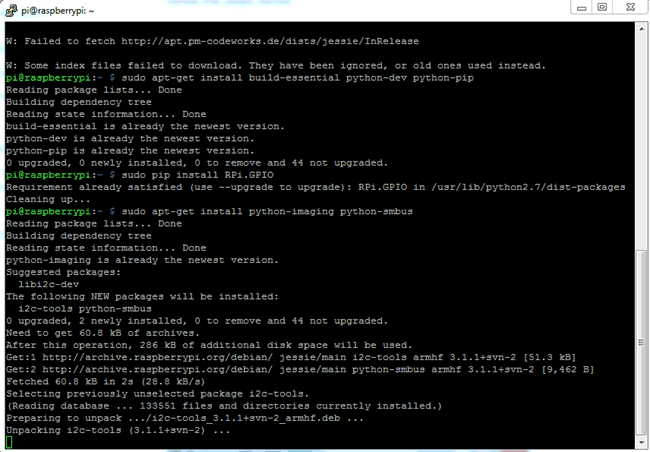Step 5: install the Adafruit SSD1306 python library

Now its time to install the Adafruit SSD1306 python library code and examples by using given commands:

```sudo apt-get install git
sudo python setup.py install

```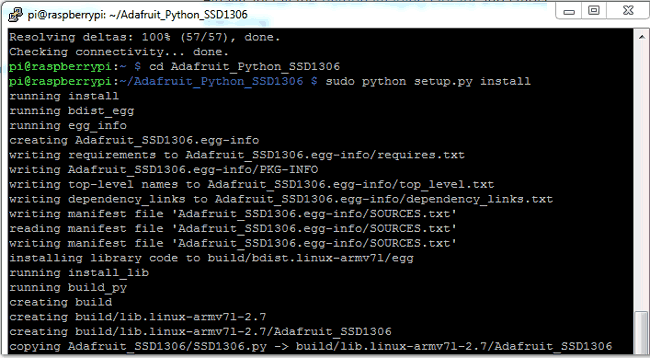Now user can find the code of OLED interfacing in Raspberry Pi and you can play directly or customize it yourself. Here we have customized a example code for demonstration. You can find full Python code at the end of the article.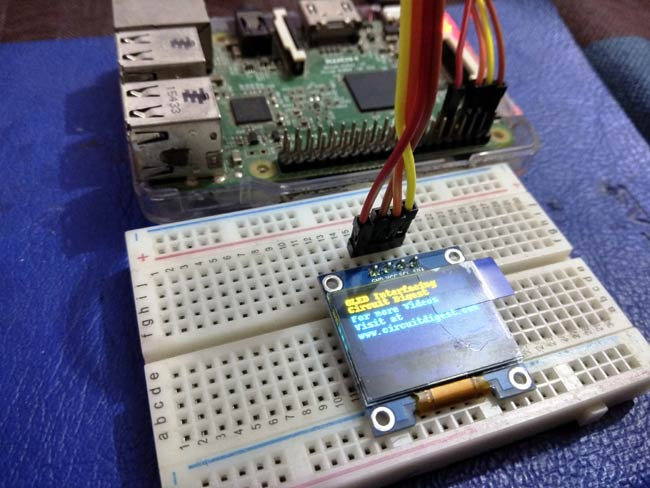### Programming Explanation:

Programming part for interfacing OLED with RPi project is easy. first, we need to import some necessary libraries.

```import time
from PIL import Image
from PIL import ImageDraw
from PIL import ImageFont
import subprocess
```

After this initialize the display

```RST = 0

disp.begin()
disp.clear()
disp.display()

width = disp.width
height = disp.height

image1 = Image.new('1', (width, height))

draw = ImageDraw.Draw(image1)
draw.rectangle((0,0,width,height), outline=0, fill=0)

x = 0

After this, we can send data or image to OLED by using given code
```# Write two lines of text.

disp.clear()
disp.display()
draw.text((x, top),       "OLED Interfacing " ,  font=font, fill=255)
draw.text((x, top+8),     "Circuit Digest", font=font, fill=255)
draw.text((x, top+16),    "For more Videos",  font=font, fill=255)
draw.text((x, top+25),    "Visit at",  font=font, fill=255)
draw.text((x, top+34),    "www.circuitdigest.com",  font=font, fill=255)

# Display image.
disp.image(image1)
disp.display()
time.sleep(2)

if disp.height == 64:
image = Image.open('img1.png').convert('1')
else:
image = Image.open('img1.png').convert('1')

disp.image(image)
disp.display()
time.sleep(2)

if disp.height == 64:
image = Image.open('img3.jpg').convert('1')
else:
image = Image.open('img3.jpg').convert('1')```

This OLED comes in two variants one is 128*32 and other is 128*64 so the user can select anyone accordingly while initializing OLED. Here we have written this code for both. The user needs to only initialize OLED for 128*64 pixel like:

```disp = Adafruit_SSD1306.SSD1306_128_64(rst=RST)
```

All the code and functions are easy to understand and no further explanation is needed. Just start and try playing with height, width & images and try some other functions to create some more cool geometric figures.

Full python code and Demonstration Video is given below and here you find the images which we have used in this program.

Also check OLED Interfacing with Arduino.

Code

import time
from PIL import Image
from PIL import ImageDraw
from PIL import ImageFont
import subprocess

RST = 0

disp.begin()
disp.clear()
disp.display()

width = disp.width
height = disp.height
image1 = Image.new('1', (width, height))
draw = ImageDraw.Draw(image1)
draw.rectangle((0,0,width,height), outline=0, fill=0)

x = 0

while True:
draw.rectangle((0,0,width,height), outline=0, fill=0)

# Write two lines of text.
disp.clear()
disp.display()
draw.text((x, top),       "OLED Interfacing " ,  font=font, fill=255)
draw.text((x, top+8),     "Circuit Digest", font=font, fill=255)
draw.text((x, top+16),    "For more Videos",  font=font, fill=255)
draw.text((x, top+25),    "Visit at",  font=font, fill=255)
draw.text((x, top+34),    "www.circuitdigest.com",  font=font, fill=255)

# Display image.
disp.image(image1)
disp.display()
time.sleep(2)

if disp.height == 64:
image = Image.open('img1.png').convert('1')
else:
image = Image.open('img1.png').convert('1')

disp.image(image)
disp.display()
time.sleep(2)

if disp.height == 64:
image = Image.open('img3.jpg').convert('1')
else:
image = Image.open('img3.jpg').convert('1')

disp.image(image)
disp.display()
time.sleep(2)

if disp.height == 64:
image = Image.open('img4.jpg').convert('1')
else:
image = Image.open('img4.jpg').convert('1')

disp.image(image)
disp.display()
time.sleep(2)

Video